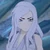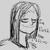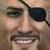24,888 Pages

## Step 1: Find the Size of the Explosion

First, you have to find the exact page, or scene where an explosion is shown as a feat. Depending on the environment where the explosion occurred, you may have to scale the size of a smaller object that is shown in the map. Let's do an example...

### Example 1: An Explosion Occurs

An explosion of unknown size occurs in this picture, engulfing the object. Find the size of the explosion. The explosion is shown in the below scene.

• The first thing that notice is that you do not know the exact size of the object shown in the picture.
• However, you are allowed to find the size of the object by comparing it with an another object, such as the one shown beside the object.

## Step 2: Finding the Radius of the Explosion

From example 1, there is a scene that shows the object in relation to the object shown from the first picture. This is located below. We will use the height of the door as a reference in this case.

• Given that the door size of the door is 7 feet, or 2.13 metres tall, you can now find the size of the object.
• Log down the size of the object.
• After you have found the size of the object, you compare the size of the object to the diameter of the explosion shown in the first picture.
• Divide the diameter by two to get the radius of the explosion.

## Step 3: Finding the Yield of said Explosion

Here is a sample calculator that you can use in order to find the yield of an explosion, provided that you have found the exact diameter of it. Keep note that if the explosion is against a surface it is preferred, but not required, that you use the surface detonation setting on this calculator.

• Generally, you take the near-total fatalities section from the list of calculated values/calculator above.
• As for why it is used, the definition of near-total fatalities means states that: all large above-ground structures are destroyed within that radius, as well as causing 100% fatalities to anyone that is affected within the radius due to sheer pressure alone.
• When you get the result of said explosion, you multiply the value by 0.5. This is because only 40 to 50 percent of the total energy of the explosion is actually from the blast.
• Keep in mind that if the explosion is an actual nuclear explosion, you can disregard the point above.

If you are having a hard time using the nuclear calculator, this equation can be used to find the yield of said explosion.

• Air blast radius (near-total fatalities) Yield: Y = ((x/0.28)^3)/1000 with Y in megatons of TNT and x the radius in km.

## Durability From Tanking an explosion

Let's say an explosion occurs and a character endures the explosion. If that explosion doesn't occur close to the character the amount of energy that hits it will be significantly lower than the full energy of the explosion. To find the actual amount of the energy from the explosion that he/she tanks one first applies the inverse square law.

What does the inverse square law state?

• The further you are away from an explosion, the less damage you will take from it. That is, because the power of said explosion is distributed over a larger and larger surface area as the distance from the epicenter increases.
• The surface area of a sphere is A = 4πr^2. This is relevant because when an explosion occurs, the energy of said explosion radiates outwards as a sphere, unless obstructed. If it happens on the ground the explosion is restricted in the downwards direction and hence only expands in half a sphere, meaning A = (4πr^2)/2. Other surface areas are imaginable as well.
• The intensity of said explosion at a distance of r is I = P/A, where I = Joules of energy, A is as above and P=Power/Yield of explosion, in Tons of TNT.
• I is a measure of Energy per Area (J/m^2 in SI units).

How does that relate to the durability needed to survive an explosion?

• The amount of energy that hits a target if r meters away from an explosion is E=I*CA, where I is calculated as described above and CA is the area of the cross section of the target (the cross section orthogonal to the direction the explosion expands into, to be specific) and E is the energy the target is hit by.
• CA is approximately 0.68 m^2 for a grown human. It can also be estimated as half of the bodies surface area calculated using this, but that is a slight overestimation.

Let's do an example.

### Example

An omnidirectional explosion of 7 kilotons of TNT occurs, and a human 30 meters away from the epicenter endures the explosion. How high is the durability of the human?

• First, we set P = 7000 Tons of TNT, as that is the yield of said explosion.
• Second, we set the radius, or r = 30m
• Third, we find the value of I, or the intensity of the explosion at a specific distance.
• I = (7000 Tons of TNT) / (4π((30m)^2))
• This means at 30 metres away from the epicenter of the explosion, the shockwave is hitting with an intensity of I = 0.619 Tons of TNT per m^2.
• CA = 0.68 m^2 for a human.
• So I*CA = 0.619 Tons of TNT per m^2 * 0.68 m^2 = 0.42092 Tons of TNT = E.
• The character can withstand a 0.42092 Tons of TNT blast, meaning Building level durability.

## Start a Discussion Discussions about Explosion Yield Calculations

•#### New Way to Calc Pillar Explosions (Brought to You By Dragon Maid)

5 messages
•Bump
•Bump
•#### A minor thing about explosions

2 messages
•Nothing too big here, but, something I've been thinking about for a little bit, wouldn't measuring height of an explosion count...
•interesting
Community content is available under CC-BY-SA unless otherwise noted.